# Trees - orchard

The 3/5 trees are apple trees and 1/3 of the trees are cherries. The remaining 5 trees are pear trees. How many trees are in the orchard.

n =  75

### Step-by-step explanation:

3/5n + 1/3n + 5 = n

3/5•n + 1/3•n + 5 = n

n = 75

Our simple equation calculator calculates it.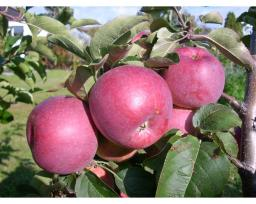Did you find an error or inaccuracy? Feel free to write us. Thank you!Tips to related online calculators
Need help to calculate sum, simplify or multiply fractions? Try our fraction calculator.
Do you have a linear equation or system of equations and looking for its solution? Or do you have a quadratic equation?

## Related math problems and questions:

• Orchard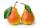Three-fifths of the orchard are apple trees, one-third of the trees are cherries. The remaining 5 trees are pears. How many trees are in the orchard?
• Orchard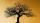3/5 of the trees in the orchard are apples, 1/3 are cherries and the remaining five trees are pear. How many trees are in the orchard?
• Trees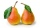Two-fifths of the trees in the orchard are apple trees, one-sixth a pear. The remaining 26 trees are cherries. How many pears are in the orchard?
• Trees3/5 trees are apples, cherries are 1/3. 5 trees are pear. How many is the total number of trees?
• Proportion 3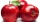For every 8 mango trees in the orchard, there are 4-star apple trees. If there are 1320 trees, how many trees of each kind are there?
• Orchard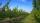10 trees in 5 lines grows in the orchard. How many trees are in the orchard?
• TreesIn the orchard, 38 apple trees and 20 pear trees grew. How many trees there grow?
• An orchard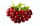In an orchard, there are 28 apple trees 27 orange trees and 14 cherry tree. What is the ratio of apple trees to cherry trees?
• There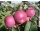There are 32 trees of three species in the orchard. 25% cherries, three eighty pears, others plums. How many plums are in the orchard?
• Cakes1/3 poppy cake, 1/3 apple, 15 pieces of cheese. How many are totally cakes?
• Fruit equation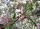Find how many peaches could be bought instead of buying one melon, one pear, one apple and two plums if we know that: • Melon and plum cost the same as three apples • if we subtract the price of a pear from the price of peach, we get the price of plum • T
• TreesFrom the total number of trees in the orchard, there are two-fifths pearls and apples are three eighty. The rest of the trees are 9 ceremonial. How many trees are in the set?
• OrchardOne-eighth of the trees in the fruit plant in winter froze, and one-twelfth of damaged disease and pests. Healthy trees remained 152. Is it enough to supply 35 trees to restore the original number of trees in the orchard?
• Lenka and money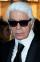When Lenka bought pants from 1/3 of her savings shirt from 1/5 and 1/8 spent at the hairdresser, she was left 328 Kč. How much money was saved? How many hairdressers cost?
• Trip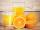On the trip drank 3/10 of pupils tea, 2/5 cola, 1/4 mineral water and remaining 3 juice. How many students were on the trip?
• Bag of peanuts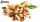Joe eat 1/3 of a bag of peanuts, mark eat 1/4 of the remaining in the bag of peanuts, Alvin eat 1/2 of the remaining bag of peanuts, peter eat 10 peanuts, there are 71 peanuts left. Hon many peanuts were in the bags?
• In the orchardIn the orchard, they planted 25 apple trees, 20 pears, 15 plums and 40 marbles. A strong late frost, however, destroyed a fifth of all new trees. Unfortunately, it was all the trees of one kind of fruit. What is the probability that the plums have died ou